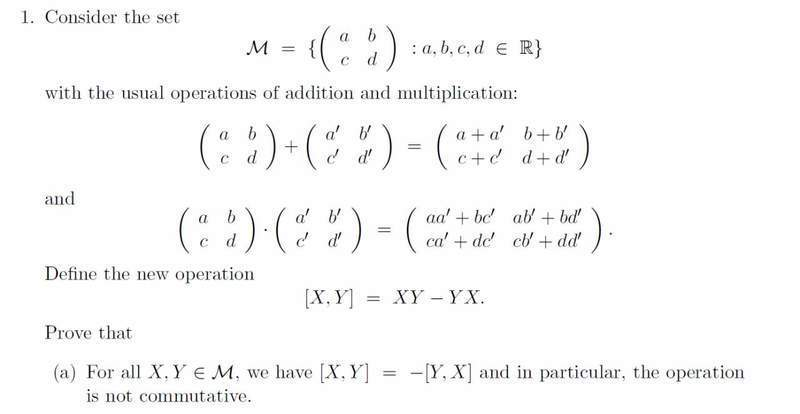# Proofs with Matricies

joedozzi

## Homework Equations## The Attempt at a Solution

-(y, x) = -(YX-XY)
= XY-YX

Can I do this or would I have to define a matrix X= ( a b c d ) Y= ( e f g h)

And prove it that way? I am just really confused

Mentor

## Homework Equations

View attachment 50857

## The Attempt at a Solution

-(y, x) = -(YX-XY)
= XY-YX

Can I do this or would I have to define a matrix X= ( a b c d ) Y= ( e f g h)

And prove it that way? I am just really confused

What you have above would work, but your notation is awful! Try to use the notation as given in the problem. Also notice that uppercase letters represent matrices, and lowercase letters represent the entries in a matrix.

Assuming that matrices X and Y are in M, then [X, Y] = ?
Keep working with the expressions you get until you end up with -[Y, X].

joedozzi
[X, Y]= YX- XY
Thus [Y, X]= XY- YX
then -[Y, X]= -(XY- YX)
then -[Y, X]= -XY +YX

Like that? So i don't have to use matricies with variables, just use X and Y to represent a matrix?

Mentor
[X, Y]= YX- XY
Thus [Y, X]= XY- YX
then -[Y, X]= -(XY- YX)
then -[Y, X]= -XY +YX

Like that? So i don't have to use matricies with variables, just use X and Y to represent a matrix?

This is easier to follow.

[X, Y] = XY - YX = -(YX - XY) = -[Y, X]

Can you say why each pair of successive equal expressions is valid?

joedozzi
Properties of Matricies?

joedozzi
And Thanks your honestly a huge help!

Mentor
Properties of Matricies?
That's pretty vague. Also, there are a number of steps. One reason doesn't fit them all.